# Atmospheric loss and supply by an impact-induced vapor cloud: Its dependence on atmospheric pressure on a planet

## Abstract

Hypervelocity impact would vaporize the impactor and part of planetary surface and create a rock vapor cloud. Results from previous studies suggest that the energetic impact would have a role to blow off and cause a large-scale loss of the planetary atmosphere through expansion of the vapor cloud. Impact also has been considered as a material source. Numerous, repeated impact events during the heavy bombardment period could greatly affect the amount of volatiles and the atmospheric pressure on the planetary surface in either way. To discuss the evolution of the atmospheric pressure by impacts, we carried out hydro-calculations with a two-dimensional hydrodynamic code and investigated the dependence of the loss and supply of the atmosphere on the atmospheric pressure. We integrated both effects by impacts over impactor size distribution and assessed the evolution of the atmospheric pressure on early Mars. Using this approach, we found that the numerous impacts likely increase the atmosphere monotonically or control the atmospheric pressure to some value, rather than causing the monotonic decrease as the previous study suggested.

## 1. Introduction

The presence of numerous craters on the Moon and Mars suggest that the terrestrial planets experienced intense series of impact events after the main stage of their formation. The dating analysis of lunar rocks suggests that a ‘heavy bombardment period’ occurred about 3.8 billion years ago, when the terrestrial planets had already grown to nearly their present size. At this time, impact velocity would be high enough to vaporize fully or partially the impactor and part of the planetary surface. Such energetic impact events could affect the volatile budget on the planet.

Cameron (1983) suggested the possibility that impacts would cause the loss of planetary atmospheres: hypervelocity impact events create cloud of rock vapor that could blow off a fraction of the planetary atmosphere via energetic expansion of the vapor cloud. The process is referred to ‘impact erosion’. Melosh and Vickery (1989) analytically estimated the condition for the large-scale loss of the atmosphere and integrated effects of the atmospheric loss by impacts over impactor size distribution. Their results suggested that the atmospheric pressure would significantly decrease through the heavy bombardment period on Mars. The atmospheric mass eroded from the planet has been extensively studied and revised by several groups with more sophisticated approaches: an analytical model in Vickery and Melosh (1990) and hydrodynamic calculations of vapor expansion in Newman et al. (1999). Recently, Shuvalov and Artemieva (2002) performed full simulations for impacts of asteroids and comets on the present-day Earth. In these three papers (Vickery and Melosh, 1990; Newman et al., 1999; Shuvalov and Artemieva, 2002), however, the calculations are carried out only under the current atmospheric pressure on the Earth, namely, 1 [bar] air. It is not enough to discuss the atmospheric pressure change through the bombardment because the atmospheric pressure may greatly change (up to two orders of magnitude) by impacts through the heavy bombardment period on Mars, as discussed by Melosh and Vickery (1989). The change in the atmospheric pressure could, in turn, affect the loss efficiency of the atmosphere by impacts.

Impacts also contribute to the supply of atmospheric volatiles. A fraction of the volatiles in the asteroids and comets does not escape and is retained on the planet. Also, there may be volatiles buried in the planet that are liberated but do not escape. The competition between the atmospheric loss by vapor expansion and the volatile supply by retention of the vapor cloud would affect the volatile budget. The mass lost from the planet and its atmospheric pressure dependence, however, has not been fully investigated by hydrodynamic calculation. Melosh and Vickery (1989) did not account for the supply of volatiles, while Chyba (1990) and Zahnle (1993) assumed the rock vapor to be totally retained on the planet in their discussion of the volatile budget on Earth and Mars, respectively. To discuss the influence in volatile budget by impacts, it is necessary to consider the effects of both the atmospheric blow-off and vapor retention, and their atmospheric pressure dependence.

In this paper, we develop a two-dimensional (2-D) hydro-dynamic code and carry out calculations of vapor expansion over a wide range of atmospheric pressure on a planet to investigate the pressure dependence of the behavior of the atmosphere and vapor cloud. Our hydrocode is equivalent to that by Newman et al. (1999) and may be rather primitive compared with the full simulations in Shuvalov and Artemieva (2002). However, it enables us to carry out calculations over the wider parameter range because of the less computational load. Also, our simple model setting helps us to understand the physics which controls the induced flow and the mass of the eroded atmosphere and retained vapor cloud. We integrated the impact-induced loss and supply of the atmosphere over a plausible impactor mass distribution for Mars, taking the derived atmospheric pressure dependence into consideration. Our results indicate that the integrated effect of numerous impact events is to monotonically increase or to control to some value the atmospheric pressure on Mars during the heavy bombardment period, instead of the monotonic decrease suggested by Melosh and Vickery (1989).

## 2. Numerical Method

### 2.1 Model setting

An expansion of the impact-induced vapor cloud is considered in a plane-parallel atmosphere (Fig. 6(a)), and both the vapor and the atmosphere are assumed to be ideal gas. The atmosphere is gravitationally bound to the planet. We assume that the atmosphere is stratified isothermally, which gives the exponential distribution of the atmospheric pressure and density with respect to altitude z: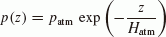(1)

and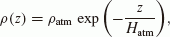(2)

where patm and ρatm are the pressure and density of the atmosphere at the planetary surface (z = 0), respectively, and Hatm is its scale height. The vapor cloud, which is produced by shock heating around the impact point, is assumed to be uniformly distributed within a hemisphere of radius rvap centered at the impact point. We assume that the vapor cloud is initially at rest, so that the initial energy of the vapor is entirely thermal. We assume adiabatic flows and neglected radiative and conductive heat transport. The specific heat ratio γatm of the atmosphere is fixed as 1.4 in this paper. The ratio γvap of the vapor cloud depends on various chemical reactions and ionization in the vapor cloud and is not well constrained yet. Fitting of the decay rate of impact flash intensity gives the estimate of the specific heat ratio of 1.216–1.250 for pumice (Davis, 2009). We assumed that γvap equals 9/7(1.29), which is the same value as the one used in Vickery and Melosh (1990).

### 2.2 Formulation and computational model

In the above model setting, the driven flow of the vapor and atmosphere is to be axially symmetric. We developed a hydrodynamic code in a 2-D cylindrical coordinate system to calculate the flow. We used the hydrodynamic equations expressing mass, momentum and energy conservation: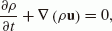(3)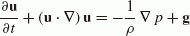(4)

and(5)

where γ and g are the specific heat ratio of the gas and the gravitational acceleration of a planet, respectively. Boldface symbols in the text and equations denote vector quantities. Our code is based on the algorithm CIP (Cubic Interpolated Propagation: Yabe and Aoki, 1991; Yabe et al., 1991). The CIP scheme is a kind of semi-Lagrangean finite difference scheme. In this scheme, the hydrodynamic equations (3)(5) are split into two phases, the advection phase and the non-advection phase, with respect to the physical values (ρ, p and u) and their spatial derivatives. The advection phase is solved by propagating an upstream profile which is constructed inside the grid cell with a cubic polynomial. This scheme can solve hyperbolic equations with third-order accuracy in time and space and capture a sharp shock wave very well with the smaller grid number and less diffusion. The feasibility of this method has been demonstrated by applying it to various fluid flow problems, such as laser-induced evaporation and vapor expansion (Yabe et al., 1995; Ohkubo et al., 2003) and shock wave generation (Takewaki and Yabe, 1987). In the field of planetary science, the break-up of Shoemaker-Levy 9 entering the Jovian atmosphere was studied with this method (Yabe et al., 1994). Our code was tested with two typical strong-shockwave problems: a 1-D shock-tube problem and the Sedov blast wave problem. Compared with the analytic solution of the 1-D shock-tube problem, the relative errors are 4.9% for the velocity, 3.5% for the pressure and 5.2% for the density.

We normalized the hydrodynamic equations and initial conditions by introducing appropriate scales of length, velocity and pressure. We took the initial vapor radius rvap, sound speed cvap in the initial vapor cloud and the atmospheric pressure patm at the surface as the scales. We then derived the four dimensionless parameters, which are defined as follows,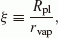(6)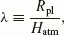(7)(8)

and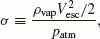(9)

where Rpl and Vesc are the planetary radius and escape velocity, respectively, evap and ρvap are the specific energy and the density of the initial vapor cloud.

The CIP scheme requires artificial viscosity terms in the momentum and energy conservation equations to capture sharp shock waves. The non-dimensional equations including the artificial viscosity are(10)

and(11)

where the tilde denotes a normalized variable. The reduced gravity accelerationof the planet is written using the non-dimensional parameters:(12)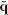is the artificial viscosity, and subscript i should be read r in r-direction and z in z-direction. The artificial viscosity in each direction is written as(13)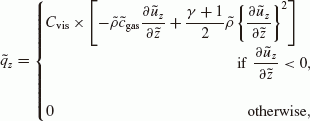(14)

where Cvis is the viscosity coefficient and Cgas is the non-dimensional sound speed of the gas. In our calculations, Cvis is set at 0.75. The boundary between the vapor cloud and the atmosphere is captured by solving the following advection equation with respect to the tracer field ø,(15)

where the value of ø is 0 for the atmosphere and 1 for the vapor cloud. Thus, the flow is controlled by the four dimensionless parameters and the specific heat ratios.

We used a staggered grid system with the finite difference scheme. The initial vapor cloud is resolved by 10 × 10 regular zones. For the other region, the spatial grid interval is set to increase by a geometric series in which the ratio of successive terms is 1.05. The entire computational region is resolved into 180 × 180 rectangular zones and may become as large as about 1 × 108 times the initial vapor size. In the case that the ratio of successive terms is 1.02, the mass of the atmospheric and vapor loss increases by about 4%. We applied solid boundary conditions to the planetary surface and z-axis and non-reflective boundary conditions to the edges of the atmosphere. The time interval is determined at each time step from the Courant-Friedrichs-Lewy (CFL) condition. We set the CFL number at 0.2.

## 3. Impact Erosion and Supply

### 3.1 Definition of the “blown-off mass” for the atmosphere and vapor cloud

We assume that gas whose root-mean-square velocity exceeds the escape velocity of the planet would be escaping from the planet. Figure 1 shows the time variation of the escaping mass of the atmosphere and vapor cloud, which is computed on this assumption. A strong shock wave in the atmosphere, which is induced by the explosive vapor expansion, propagates away from the impact point at higher velocity than that of the vapor expansion. The preceding shock wave and/or the further expansion of the vapor cloud accelerate the ambient atmosphere. As a result, the escaping mass of both the atmosphere and the vapor cloud rapidly increases at the early stage. Thereafter, both the mass of escaping vapor and the mass of escaping atmosphere level off and approach asymptotic values (Fig. 1). We defined these final escaping masses as “the mass of the atmospheric and vapor cloud loss”, respectively.

In Fig. 1, our result is plotted with numerical result reported in Newman et al. (1999). From Fig. 1 we can see that their computation time is not enough for the escaping mass to level off. It is possible that these researchers calculated the flow at the very early stage, during which the shock wave plays a dominant role in atmospheric acceleration. In such an early stage, a larger portion of the vapor cloud, which has most of the momentum, remains near the planetary surface. Also, our calculation shows that the escaping vapor cloud accounts for a large fraction of the total escaping mass. Though they did not distinguish the escaping vapor from the escaping atmosphere, it is speculated that the total escaping mass in Newman et al. (1999) mainly represents the escaping mass not of the atmosphere, but of the vapor cloud.

### 3.2 Atmospheric-pressure dependence of the vapor-cloud loss

We derived the mass of the vapor-cloud loss for the various atmospheric pressures (σ−1) (Fig. 2) for three different initial vapor radii (ξ). The other parameters λ and ε, which correspond to the atmospheric scale height and the specific energy of the initial vapor cloud, are fixed through the calculations in this plot. In order to expand and escape, the vapor cloud needs to give more momentum to the more massive atmosphere. Therefore, more vapor cloud is retained as the atmospheric pressure increases at large atmospheric pressure. On the other hand, under low atmospheric pressure, the vapor cloud can escape freely. In such situation, it is a striking feature that the mass fraction of the vapor loss is almost independent of the vapor radius (mass). The maximum mass fraction of the vapor loss, which occurs at the limit of low atmospheric pressure, greatly changes with the specific energy of vapor cloud and is about 0.6–0.7 for ε = 2.0 and 0.01–0.02 for ε = 0.5(ε is the dimension-less parameter associated with the specific energy of vapor cloud, see Eq. (8)).

The vapor mass retained on the planet is derived from subtracting the mass of its loss from its total mass. The results indicate that the vapor mass retained on the planet is almost proportional to the vapor mass but independent of the ambient pressure at the limit of minimum retention, and that the supply of the volatiles would be more effective for the larger vapor mass.

### 3.3 Atmospheric-pressure dependence of the mass of atmospheric loss

We investigated the atmospheric pressure dependence of the atmospheric mass eroded by the vapor expansion for three different initial vapor cloud radii (Fig. 3). The other parameters λ and ε are fixed through the calculations in this plot as well as in the plot for the vapor cloud loss. The pressure dependence shows different trends at the low- and high-pressure regimes. For the lower atmospheric pressure, the mass fraction of the atmospheric loss can be approximated by a power law distribution of the non-dimensional pressure σ−1. In this regime, the power-law exponent is about −0.7 for any initial vapor radii (mass). Considering that the total atmospheric mass on the planet is proportional to the surface atmospheric pressure, this means that the mass of the atmospheric loss increases with increasing atmospheric pressure to the power about 0.3. On the other hand, as the atmospheric pressure increases, more energy is required for the vapor cloud to expand and expel the atmosphere. Thus, for the larger atmospheric pressure, the power-law dependence does not hold and the mass of the atmospheric loss rapidly decreases with increases of the atmospheric pressure. This implies that the mass of the maximum loss of the atmosphere occurs at a certain atmospheric pressure, at which the pressure dependence changes. In the following, we call this pressure as the ‘optimum loss pressure’.

### 3.4 The shape of atmospheric escape region

We also found the transition in the shape of “atmospheric escape region”, where the atmosphere can escape from the planet with the atmospheric pressure, as well as in the mass of the atmospheric loss. The gray zone in Fig. 4 shows the escape region. Figure 4 shows that the shape of the escape region strongly changes with the atmospheric pressure: for large atmospheric pressure, it is cone-shaped (Fig. 4(a)), while it takes on the shape of a bowl or truncated-cone at low atmospheric pressure (Fig. 4(b)).

The shape of the escape region reflects the dynamic flow of the atmosphere. The atmosphere is strongly compressed by the passage of the preceding shock wave so that a high-pressure shell of the shocked atmosphere is formed ahead of the vapor expansion front. Since the atmospheric mass in the shell is large for a massive atmosphere, the vapor cloud is required to transfer more momentum to expand. Thus, the vapor cloud loses its momentum at the very early stage while the flow is almost radial outward from the impact point. This process results in the spherical shape of the high-pressure shell. The shape of the escape region is simply determined with the critical zenith angle θ from the vertical, which is smaller than that the vapor cloud can push away the atmosphere in the azimuthal bin, resulting in the cone-shaped escape region (Fig. 4(a)).

In contrast, the bowl- or truncated-cone-shaped escape region results from the deviation of the vapor flow from the radial direction. As the atmospheric pressure decreases, the vapor cloud can expand further against the ambient atmosphere. The velocity of the shock wave is zenith angle-dependent in the stratified atmosphere: that is, the higher the velocity, the smaller the zenith angle. The velocity differences between the zenith angles changes the shape of the high-pressure shell from the closed spherical shape to an open bowl or truncated-cone profile, and the atmosphere and vapor cloud within the shell flows along the shell wall. As a result, the deflection of the radial-vapor flow induces the arc-like flow in the atmosphere with the large zenith angle (near surface), which results in the truncated-cone shaped escape region (Fig. 4(b)).

The shape of the escape region allows us to speculate on the pressure dependence of the escaping atmospheric mass. Although the atmospheric density is proportional to the atmospheric pressure, the blown-off mass of atmosphere varies as pressure to the 0.3 power for small atmospheric pressures, as shown in Fig. 3 and the previous section. Under such an atmospheric pressure, the escape region is truncated-cone-shaped, and its bottom radius determines the mass of the atmospheric loss. The vapor cloud can expand further against the lower atmosphere and, as a result, the bottom radius of the escape region spreads out. This compensates for the increase in atmospheric density with atmospheric pressure and weakens the sensitivity of the blown-off mass to the pressure.

### 3.5 The optimum loss pressure and an empirical formula of the eroded atmospheric mass

The typical value of the optimum loss pressure obviously depends on the other parameters, such as vapor specific energy. We performed calculations for the various λ and ε and found that the power-law dependence appears while the atmospheric pressure satisfies the following condition: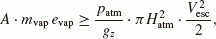(16)

where mvap is the mass of the vapor cloud and A is an empirical non-dimensional constant (about 1–3 hundredths). The right-hand part of the equation denotes the energy necessary to accelerate the atmospheric mass within a column with the bottom radius Hatm to the escape velocity Vesc. Consequently, the inequality (16) means that the pressure dependence changes if the vapor energy exceeds a few tens to about one hundred of times the energy required for blowing off the atmosphere in the column. Since the optimum loss pressure is the pressure at which the pressure dependence changes, equality in Eq. (16) gives the optimum loss pressure.

Melosh and Vickery (1989) assumed the two criteria from physical consideration for the loss of all the atmosphere above the tangent plane to the impact point. Their criteria are that (1) the expanding velocity of the vapor cloud exceeds the escape velocity of the planet, and that (2) the mass of the vapor cloud exceeds the mass of the atmosphere above the tangent plane. From these criteria, the following inequality should be satisfied;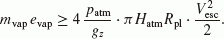(17)

Here, we used the same relation between the impact and created vapor condition as assumed in Melosh and Vickery (1989) (Eqs. (19) and (20) in this paper). The reader should note that the ‘specific energy of the vaporized material’ in these reseachers’ paper corresponds to the sum of our specific energy of the vapor cloud and the specific energy of the vaporized material. Compared with the inequality (17), one can find that our inequality (16) associated with the optimum loss pressure can be derived up to constant factors by replacing Rpl in the inequality (17) with Hatm.

We derived the empirical formula on the relation between the atmospheric mass eroded from the planet and the other parameters, such as the atmospheric pressure and the vapor radius, using a function a of λ and ε:(18)

Please note that the empirical formula (18) is valid only when the relation (16) holds.

### 3.6 Comparison with the hemispheric blow-off model

We also applied the hemispheric blow-off model in Vickery and Melosh (1990) to calculate and compare their pressure dependence (Figs. 2 and 3). The pressure dependence is in remarkably agreement with our hydrodynamic calculations for low atmospheric pressure. However, at some large pressures in the hemispheric blow-off model, both the loss of the atmosphere and vapor cloud rapidly decrease and shut off, while our results show more gradual decreases. The difference results from the difference in the criteria for escape from the planet. In the model of Vickery and Melosh (1990), it is required that the radial velocity averaged in the azimuthal sector exceeds the planetary escape velocity to blow off the gases. In actual conditions, however, radial velocity distribution exists, and the front of the expanding vapor cloud is faster than that of its most inner portion. The criterion using the averaged velocity in the sector therefore underestimates atmospheric and vapor loss by the impacts.

The mass of the atmospheric loss estimated by Vickery and Melosh (1990) is less than that of our hydrodynamic calculations by some factor, while the pressure dependence is in good agreement between both models. For the parameter set used at the calculations in Figs. 2 and 3, the factor is almost 0.5. Although the value of the factor varies with the parameter sets, it is found that the value stays below unity over the ranges of the parameters considered.

### 3.7 The implication to the atmospheric pressure change on early Mars

The atmospheric pressure dependence derived from our calculations can be summarized as follows. The eroded mass increases with the power-law dependence against the atmospheric pressure, while the retained fraction of the vapor cloud is nearly constant, when the impact satisfies condition (16), which means the impact is energetic enough against the ambient atmospheric mass. If the impact is less energetic, the eroded mass of both the atmosphere and vapor cloud rapidly decreases with the atmospheric pressure and finally becomes zero.

How does the difference in the pressure dependence between the atmosphere and vapor cloud affect the volatile budget on the planet? Here, we will qualitatively discuss the atmospheric pressure change by asteroidal impacts on Mars, taking into account the derived atmospheric pressure dependence as well as the impactor-size distribution. We assumed the same relation as that used by Vickery and Melosh for the impact condition (impact velocity υimp and impactor mass mimp) and the induced vapor condition (evap and mvap) for the asteroidal impacts,(19)

and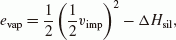(20)

where ΔHsil is the specific vaporization energy of silicate and fixed to 2.0 × 107 [J/kg]. We considered two cases for the impact velocity υimp: 14.5 [km/s] and 19 [km/s] (Fig. 5). The atmospheric scale height is fixed to 11 [km]. The impact flux during the heavy bombardment period is estimated from the lunar impact records. It is assumed that the mass spectrum obeys a power law,(21)

where f(t) is a function of time t, and, in this paper, β is fixed to −0.47 (Melosh and Vickery, 1989).

The total mass of the eroded atmosphere and of the retained vapor cloud, which is a function of atmospheric pressure and impact velocity, is written as the integral over impactor mass distribution. The impactor mass for the onset of the atmosphere and vapor cloud loss is assumed to be midep, under which the equality in the inequality (16) holds with the impactor-vapor mass relation (19) and the velocity-energy relation (20). midep is defined as: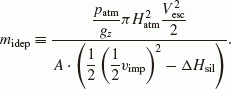(22)

We adopt 1.5 × 0 118 [kg] as the maximum impactor mass mimax, as used in Chyba (1990). Then, the total blown-off mass is given, respectively, by the integration of the mass of the retained vapor cloud (mvap_ret) and the eroded atmosphere (matm_esc) over the impactor mass distribution,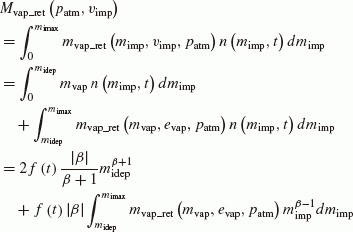(23)

and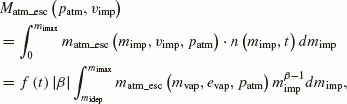(24)

where n (nimp, t) is the number of impacts between mimp and mimp + dmimp and given by(25)

The mass is given by the empirical formula (18) forthe atmospheric loss and by the interpolation from the calculation results for the vapor cloud retention, respectively. In this paper, we treated the planetary surface as a planeparallel one. The planetary surface and atmosphere, however, have a curvature, and this would be more important for the larger impactor. The assumption of the plane-parallel atmosphere causes an over estimation of the mass of the atmospheric loss because in the plane-parallel model the infinite atmospheric mass is assumed on the surface. To avoid this overestimation, we set the upper limit for the atmospheric mass eroded from the planet by the atmospheric mass above the tangent plane to the impact point.

Although most of the fraction of the retained vapor would condense with expansion and be removed from the atmosphere, some volatile elements would remain in the gas phase, leading to the increase of the atmospheric mass. We treated its supply efficiency as a free parameter, w, which denotes the mass fraction of the gas supplied to the atmosphere after the cooling of the vapor cloud. Although the parameter w is not well constrained at present, it may be inferred from the mass fraction of carbon dioxide supplied in the impactor. Carbon content is 0.03–0.05 for CI chondrites (Kerridge, 1985) and 0.001–0.002 for ordinary chondrites (Jarosewich, 1990). These values correspond to 0.11–0.18 and 0.04–0.07 of carbon dioxide in mass fraction, respectively, if simply assumed that the all the carbon becomes carbon dioxide.

The change of the atmospheric massas a function of the atmospheric pressure on Mars is plotted on Fig. 5. Figure 5 shows that the net change of the atmospheric mass by the impacts is normalized with the total impactor mass,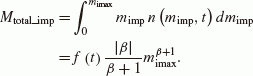(26)

The positive value of Δ a in Fig. 5 denotes an increase in the atmospheric mass and the negative one denotes a decrease. At the atmospheric pressure where the value of Δ a equals 0, the loss and supply are in balance so that the atmospheric pressure keeps its value. The results in Fig. 5 suggest two possible ways in which the atmospheric pressure evolves by impacts. One is the monotonic increase, and the other is the regulation to a certain pressure.

The monotonic increase occurs at relatively large w and/or very large atmospheric pressure. At a large w value the curve has no intersection with the x-axis, such as the case with w = 0.03 in Fig. 5(b). In this case, the volatiles supplied by impacts overwhelm the atmospheric loss, and the impacts increase the atmospheric mass on the planet regardless of its atmospheric pressure. At very large atmospheric pressure, the curve has an intersection at which the value of Δ a changes from the negative to positive with increasing atmospheric pressure, although such a large atmospheric pressure is out of range in Fig. 5. Then, the atmospheric mass would monotonically increase if the atmospheric pressure is larger than the value at the intersection.

The regulation of pressure occurs in those cases when curves in Fig. 5 intersect with the x axes at the point where the sign of Δ a changes from a positive to a negative value with increasing atmospheric pressure. (For example, the curves with w = 0.01−0.1 in Fig. 5(a) and w = 0.001 and 0.01 in Fig. 5(b)). We refer to the atmospheric pressure at such an intersecting point as pctl. Let us now consider the behavior of the atmospheric pressure by the impacts around pctl. If the atmospheric pressure is less than pctl, the value Δ a is positive so that the atmospheric pressure increases and approaches pctl by the impacts. One can have a similar result for the opposite case. This indicates that the impacts would play a role in controlling the atmospheric pressure to approach to pctl, not unilaterally to take it away.

This behavior is a natural result of the difference in the pressure dependence between the atmospheric loss and the retained vapor cloud. The eroded mass of the atmosphere shows the power-law dependence (Eq. (18)) to the atmospheric pressure and has the maximum value at the optimum atmospheric pressure. On the other hand, the mass of the retained vapor cloud is almost independent of the atmospheric pressure as long as the impact is energetic enough to satisfy condition (16). As a result, the atmospheric pressure decrease by impacts, if any, has the minimum value.

It should be noted that whether the final atmospheric pressure after the heavy bombardment reaches pctl or not depends on the total impactor mass through the heavy bombardment. Also, the value of pctl and its actual existence also depend on the value of w and the vapor energy (the impact velocity). We treated the maximum impactor mass mimax as an independent variable. Assuming that the Poisson distribution can be applied to the number of the largest impacts and that the expected value of the number of the largest impact is equal to 1, the value of mimax could vary from 0.651 × 1018 [kg] to 2.16 × 1018 [kg] by using 1σ, and then the value of pctl could change by a factor of about 2. This change is mainly caused by the change in the retained vapor mass because the mass of the retained vapor cloud is approximately proportional to mimax, while the change of the mass of the atmospheric loss is relatively less, especially under the smaller atmospheric pressure.

Zahnle et al. (1992) also considered the competition between impact erosion and supply of atmospheres over Titan, Ganymede and Callisto. They found two regimes of atmospheric evolution; the erosive regime and the accumulative regime. The erosive regime seems to be corresponding to the curves with the intersections in our Fig. 5. These authors also suggested that the equilibrium between impact erosion and supply would occur under certain conditions in this erosive regime. Their equilibrium pressure would be qualitatively equivalent to pctl in our paper. Their equilibrium pressure, however, decreases to zero with time, probably because the maximum impactor mass is supposed to decrease to zero with time in their model. The atmospheric evolution in their paper seems to have a more erosive history than that suggested by our results. Even in their accumulative regime, an initially thick atmosphere decreases its mass by the impacts. Such an erosive evolution is not only because they used quite different parameters from ours (such as the target planets, the composition and velocity of the impactor, the maximum mass of the impactor and etc.), but also because they assumed the tangent-plane mass atmospheric erosion model by Melosh and Vickery (1989), which assumes the mass of the atmospheric loss to be proportional to the atmospheric pressure. Hence, in some cases, the thicker atmosphere tends to lose the more mass and have more erosive history.

In order to discuss the time evolution and the final pressure of the atmosphere, the random impacts should be considered with Monte Carlo calculations, as Griffith and Zahnle (1995) performed, because it is plausible that the order in which each impact falls onto the planet may be one of the factors influencing the final atmospheric pressure on the planet. For example, if the largest impactor falls at the relatively late stage, the impactor would supply the enormous amount of volatiles so that the subsequent impacts cannot reduce it, and the final atmospheric pressure would be greater than pctl. As such, it would be necessary to discuss the distribution of the final atmospheric pressure.

Our results suggest that it is possible that the terrestrial planets could have the thin atmosphere under which the impact erosion is balanced against the impact supply. Especially if the initial atmosphere is thin, the planet would acquire some volatiles by the impacts and the atmospheric pressure would reach pctl. For example, Fig. 5 shows that even under the erosive conditions such that the supply efficiency w is 0.01 and the impact velocity is about 19 [km/s], Mars could get 0.03 [bar] of atmosphere with the sufficient amount of the veneer. If this happens, the composition of the atmosphere should reflect the aftermath, being similar to the volatile composition of the impactors. If Mars had lost its atmosphere by impacts, its atmospheric composition would likely modified by the volatile component in the impactors, unless they were very dry. Xe isotopic abundances in the Martian atmosphere, however, are not coincident with those of any asteroids as Zahnle indicated in his paper in 1993. It is still unknown what this means. It may suggest that Mars did not experience the intense bombardment that researchers thought or that the veneer composition was quite different from the existing asteroids-comets or very dry, volatile poor planetesimals.

## 4. Discussion

### 4.1 The effect of the wake and the initial vapor velocity

Impactor penetration accelerates the atmosphere in the entry path and creates a hot wake in which the atmospheric density is lower by a few orders of magnitude than the ambient atmosphere. The recent full-simulation by Shuvalov and Artemieva (2002) suggests that the occurrence of the wake has an important role in preventing the ambient atmosphere from escaping because the vapor cloud could go preferentially through the wake. One of their conclusions is that the difference in the shape of the induced wake contributes to more atmospheric loss by an oblique impact than by a normal impact. Unfortunately, they did not examine the mass of the vapor retained on the planet and the atmospheric pressure dependence. We therefore performed additional experiments on the effects by the wake and the initial velocity distribution within the vapor cloud. We compared the mass of the atmospheric loss for the specific cases under which they performed the numerical simulations.

For the comparison, we assumed the same impact-vapor relation used in the previous sections (Eqs. (19) and (20)) for the asteroidal impacts. For the cometary impacts, we used Eq. (27) for the specific vapor energy as used in Vickery and Melosh (1990), instead of Eq. (20),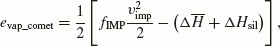(27)

where fIMP is the parameter which shows the impedance of the materials and is taken as 0.5128.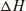is the average of the specific vaporization energy of silicate and ice, fixed to 1.15 × 107 [J/Kg].

We started our calculations from four initial conditions shown in Fig. 6 to examine the effect of the wake and initial velocity. The condition (b) is identical to the condition (a), but with a cylindrical hole set in the exponentially stratified atmosphere. The density in the hole is set to 100-fold less than the ambient atmospheric density, and its bottom radius is twofold larger than the initial vapor radius. To investigate the effects by the initial vapor velocity, we considered the other initial conditions (c) and (d). In conditions (c) and (d), the vapor has the initial velocity, such that the kinetic energy is just half the total vapor energy. The direction of the initial vapor velocity is isotropic for (c) and upward for (d). Note that the total vapor energy is the same for all conditions.

The comparison results are shown in Fig. 7. We can see that the cylindrical hole has almost no effect on the atmospheric loss, especially under the small atmospheric pressure. This is because the initial vapor cloud with the extremely high pressure would not feel the ambient atmosphere at the early stage, at least as far as under the “usual” atmospheric pressure on the terrestrial planets (up to 100 [bar]), and expand regardless of the existence and shape of the hole.

The most remarkable feature is that the direction of the initial vapor velocity could greatly change the mass of the atmospheric loss. The isotropic initial velocity produces the same results as with condition (a), in which all of the energy of the vapor cloud is given as a thermal one, while the upward initial velocity resulted in less atmospheric loss at low atmospheric pressures and more loss at its onset under the large pressure. Also, Fig. 7 shows the same power-law dependence on the atmospheric pressure regardless of the initial conditions for the non-dimensional atmospheric pressure σ−1 of less than 10−6.

These results mean that one of the key parameters for the impact-erosion problem is the azimuthal distribution of the initial vapor energy instead of its form (thermal or kinetic). The direction of the initial velocity would be different between the normal and oblique impacts. The suggestion in Shuvalov and Artemieva (2002) that the oblique impacts cause more atmospheric loss than a normal impact may be due to the difference in the direction of the initial velocity, instead of the shape of the wake. Further investigation is necessary to gain a better understanding of the effects of oblique impacts.

The comparison results show that our model with the above impact-vapor relations (Eqs. (19), (20) and (27)) predicts a greater loss of atmospheric mass than that in Shuvalov and Artemieva (2002) by a factor of 150–300 for the asteroids and 1.2–30 for the comets. The differences are more remarkable for the lower velocity impacts or the asteroidal impacts rather than the cometary impacts—both are impacts that create less vapor cloud. From the results of our preliminary experiments, which suggest that the existence of the wake is not an influential factor, the quantitative discrepancy would be primarily attributed to the impact-vapor relation that we used. The impact-vapor relation assumed in this paper (Eqs. (19), (20) and (27)) may overestimate the mass and/or the energy of the vapor cloud. Alternatively, it may be that the vapor volume is underestimated in Shuvalov and Artemieva (2002) because Pierazzo et al. (1997) reported that the vapor volume is underestimated by using the equation of state they used, ANEOS.

It should be noted that, even if our impact-vapor relation leads to an overestimation of the mass and energy of the vapor cloud, the scenario that the atmosphere decreases monotonically by impacts is less plausible because we tend to overestimate the effect of impact erosion. The atmospheric-pressure dependence that we derived would hold and the discussion about the pressure control mechanism involving pctl is valid qualitatively—unless the ambient atmosphere affects the vaporization process of the impactor and target surface by an impact.

### 4.2 Condensation of a vapor cloud

In this paper, we assumed that the vapor cloud behaves as an ideal gas. In actual fact, the vapor cloud condenses as the vapor expands and its temperature decreases. Here, we consider the effect of the vapor condensation to the mass of atmospheric loss, treating a vapor cloud as a parcel with condensed particles. With condensation, the equation of energy conservation is written as below, instead of Eq. (5) (see Appendix for the derivation of (28)),(28)

where(29)

and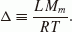(30)

c p , M m and L are the specific heat at constant pressure, the molecular weight and the latent heat of vaporization of the vapor cloud, respectively, and R is the gas constant. x is the mass fraction of the condensates. The latent heat of the impactor and target material is generally so large that the fraction x of the condensates keeps almost constant due to the release of the enormous latent heat with the condensation. Also, the value of Δ is large, and then γ p has an almost fixed value around unity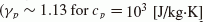, M m = 0.03 [kg/mol], L = 2 × 107 [J/kg], T = 5 × 103 [K] and x = 0.5). Consequently, the equation of energy conservation with condensation is essentially the same as that without condensation, except for the decrease in the value of the specific heat ratio of the vapor cloud γvap. To evaluate the effect of vapor condensation, we calculated the mass of the atmospheric loss with various values of γvap. We then found that the amount of atmospheric loss slightly decreases with decreasing γvap. However, the effect is no more than a factor of three even for γvap = 1.05.

## 5. Concluding Remarks

Hypervelocity impact would have great effects in the volatile budget on the planetary surface: its loss is due to the explosive vapor expansion, and its supply is due to the vapor retention. Numerous impact events during the heavy bombardment period would have the great consequences for the atmospheric evolution, especially for the atmospheric pressure change on the planets. In this paper, we performed the hydrodynamic calculations of the expansion stage of the impact-induced vapor cloud and examined the atmospheric pressure dependence of the mass that the vapor cloud would blow off and be retained, respectively. The effect of the atmospheric pressure depends on whether the impact is sufficiently energetic against the ambient atmospheric mass. If energetic enough, the mass of the blown-off atmosphere shows the power-law dependence, and the retained vapor mass is nearly independent of the atmospheric pressure. We also discussed the atmospheric pressure change by the impacts on early Mars, taking the derived atmospheric pressure dependences into consideration. We then found that the numerous impact events could change the atmospheric pressure in two possible ways: the monotonic increase or the regulation to a certain atmospheric pressure value. Which way the atmospheric pressure would evolve, however, is strongly dependent on the vapor energy and mass and the supply efficiency of volatiles in the vapor cloud. It is likely that impacts in the early stage of the terrestrial planets contribute to the supply of volatile material rather than its loss, though the quantitative discussions for the evolution of the atmospheric pressure requires more detailed full calculations, including proper EOS and the composition and content of volatile materials in impactors.

## References

• Cameron, A. G. W., Origin of the atmospheres of the terrestrial planets, Icarus, 56, 195–201, 1983.

• Chyba, C. F., Impact delivery and erosion of planetary oceans in the early inner solar-system, Nature, 343, 129–133, 1990.

• Davis, S. S., An analytical model for a transient vapor plume on the Moon, Icarus, 202, 383–392, 2009.

• Griffith, C. A. and K. Zahnle, Influx of cometary volatiles to planetary moons: The atmospheres of 1000 possible Titans, J. Geophys. Res, 100, 16907–16922, 1995.

• Jarosewich, E., Chemical-analyses of meteorites—a compilation of stony and iron meteorite analyses, Meteoritics, 25, 323–337, 1990.

• Kerridge, J. F., Carbon, Hydrogen and nitrogen in carbonaceous chondrites—Abundances and isotopic compositions in bulk samples, Geochim. Cosmochim. Acta, 49, 1707–1714, 1985.

• Melosh, H. J. and A. M. Vickery, Impact erosion of the primordial atmosphere of Mars, Nature, 338, 487–489, 1989.

• Newman, W. I., E. M. D. Symbalisty, T. L. Ahrens, and E. M. Jones, Impact erosion of planetary atmospheres: Some surprising results, Icarus, 138, 224–240, 1999.

• Ohkubo, T., M. Kuwata, B. Luk’yanchuk, and T. Yabe, Numerical analysis of nanocluster formation within ns-laser ablation plume, Appl. Phys. a-Materials Sci. Process., 77, 271–275, 2003.

• Pierazzo, E., A. M. Vickery, and H. J. Melosh, A reevaluation of impact melt production, Icarus, 127, 408–423, 1997.

• Shuvalov, V. V. and N. A. Artemieva, Atmospheric erosion and radiation impulse induced by impacts, Geol. Soc. Am. Spec. Pap., 356, 695–703, 2002.

• Takewaki, H. and T. Yabe, The cubic-interpolated pseudo particle (CIP) method: application to nonlinear and multi-dimensional hyperbolic equations, J. Comput. Phys., 70, 355–372, 1987.

• Vickery, A. M. and H. J. Melosh, Atmospheric erosion and impactor retention in large impacts, with application to mass extinctions, Geol. Soc. Am. Spec. Pap., 247, 300–389, 1990.

• Yabe, T. and T. Aoki, A universal solver for hyperbolic equations by cubic-polynomial interpolation I. One-dimensional solver, Comput. Phys. Commun, 66, 219–232, 1991.

• Yabe, T., T. Ishikawa, P. Y. Wang, T. Aoki, Y. Kadota, and F. Ikeda, A universal solver for hyperbolic equations by cubic-polynomial interpolation II. Two- and three-dimensional solvers, Comput Phys Commun., 66, 233–242, 1991.

• Yabe, T., F. Xiao, D. Zhang, S. Sasaki, Y. Abe, N. Kobayashi, and T. Terasawa, Effect of EOS on break-up of Shoemaker-Levy 9 entering Jovian atmosphere, J. Geomag. Geoelectr., 46, 657–662, 1994.

• Yabe, T., F. Xiao, and H. Mochizuki, Simulation Technique for Dynamic Evaporation Processes, Nuclear Eng. Design, 155, 45–53, 1995.

• Zahnle, K. J., Xenological Constraints on the Impact Erosion of the Early Martian Atmosphere, J. Geophys. Res, 98, 10899–10913, 1993.

• Zahnle, K., J. B. Pollack, and D. Grinspoon, Impact-Generated Atmospheres over Titan, Ganymede, and Callisto, Icarus, 95, 1–23, 1992.

## Acknowledgements

The constructive comments by K. Zahnle and an anonymous reviewer greatly helped us to improve this paper. We also appreciate the support of H. St. C. O’Neill to continue this study. This research was partially supported by the Ministry of Education, Science, Sports and Culture, Grant-in-Aid for JSPS Fellows.

## Author information

Authors

### Corresponding author

Correspondence to Keiko Hamano.

## Appendix A. The Equation of Energy Conservation of a Parcel with Condensation

### Appendix A. The Equation of Energy Conservation of a Parcel with Condensation

Here, we will derive the equation of energy conservation for a parcel with condensation (Eqs. (28)(30)). First, we will describe the chemical potential of the parcel, and then we will derive its specific internal energy with respect to pressure, temperature and mass fraction of the condensates from the partial derivatives of the chemical potential. We consider that the parcel consists of two phases (gas and condensates) and the gas phase behaves as an ideal gas. In the very rapid process such as the impact-induced vapor expansion, the size of the condensates is so small that the gas and condensates move together. It is assumed that the volume of the condensates is negligible.

For the gas phase, the equation of state and the internal energy is given by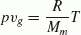(A.1)

and(A.2)

where υ and e are the specific volume and internal energy and the suffix g denotes the gas phase. M m , and R are the molecular weight, the specific heat at constant volume and the gas constant. The first law of thermodynamics, Tds = de + pdυ, gives the differentiation of entropy of gas, S g ,(A.3)

using the Mayer’s relation for the specific heat of the ideal gas. c p is the specific heat at constant pressure.

After the integration, the entropy of the ideal gas is obtained, using the entropy s g (p0, T0) at the reference state,(A.4)

From Eqs. (A.1)(A.4) and the Mayer’s relation, the specific Gibbs free energy is derived. Since we approximate the gas phase by a single component, it is equivalent to the chemical potential of the gas phase, which is defined as the partial specific Gibbs free energy(A.5)

The chemical potential of the condensates, which the suffix c denotes, is written as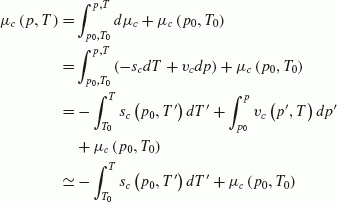(A.6)

where it is assumed that the volume of condensates are negligible in the last line of the right-hand side. Using Eqs. (A.5) and (A.6), the chemical potential of this parcel is written as,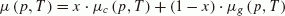(A.7)

where x is the mass fraction of the condensates and defined by(A.8)

where m is the mass in the parcel. ρ is the density of the parcel. After the partial differentiations of the chemical potential (A.7), the specific entropy and volume of the parcel are given, respectively,(A.9)

and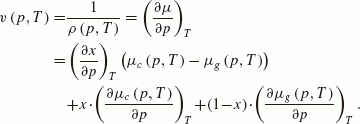(A.10)

Using Eqs. (A.7), (A.9) and (A.10), the specific internal energy is derived,(A.11)

Here, we assume that the phase equilibrium is attained at the thermodynamic state (p, T) between the gas and condensates in the parcel. By the definition,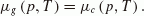(A.12)

Then, Eq. (A.11) is(A.13)

Taking the thermodynamic state (p, T) as the reference state (p0, T0), the specific energy of the parcel in the phase equilibrium is given by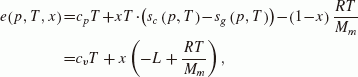(A.14)

where L is the constant latent heat of vaporization and defined by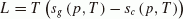(A.15)

if the phase equilibrium is attained under the thermodynamic state (p, T).

If the motion is adiabatic, the equation of energy conservation is written as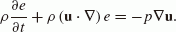(A.16)

Using Eq. (A.14) and the equation of mass conservation, we can derive the equation of energy conservation for the parcel with condensation as below,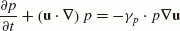(A.17)

where γ p is the effective specific heat ratio of the parcel, which is defined as(A.18)

and(A.19)

It is found that γ in Eq. (5) should be replaced with γ p in order to take into account the effect of condensation.

## Rights and permissions

The images or other third party material in this article are included in the article’s Creative Commons licence, unless indicated otherwise in a credit line to the material. If material is not included in the article’s Creative Commons licence and your intended use is not permitted by statutory regulation or exceeds the permitted use, you will need to obtain permission directly from the copyright holder.

To view a copy of this licence, visit https://creativecommons.org/licenses/by/4.0/.

Reprints and Permissions

Hamano, K., Abe, Y. Atmospheric loss and supply by an impact-induced vapor cloud: Its dependence on atmospheric pressure on a planet. Earth Planet Sp 62, 599–610 (2010). https://doi.org/10.5047/eps.2010.06.002

• Revised:

• Accepted:

• Published:

• Issue Date:

• DOI: https://doi.org/10.5047/eps.2010.06.002

### Key words

• Impact erosion
• late heavy bombardment
• Mars atmosphere
• hydrodynamic calculation